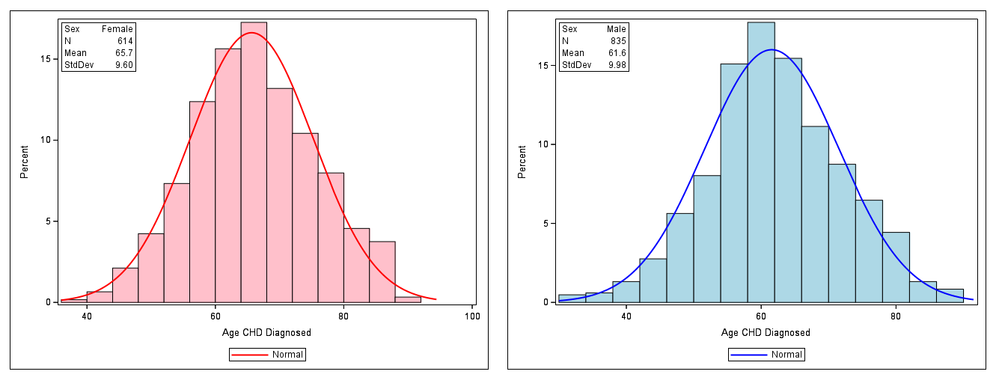## Gaussian curves in SAS

Hi all,

any idea how to create Guassian curves in sas. Any code sample?

I guess it's easy but it's new for me. My client wants that for a set of diagnosis ages in a DB (Number of cases, Mean, Standard Deviation)

Thanks

1 ACCEPTED SOLUTION

Accepted Solutions

## Re: Gaussian curves in SAS

Addendum: Here's a somewhat crude example, again using sashelp.heart:

``````proc sql noprint;
select n(agechddiag), mean(agechddiag) format=4.1, std(agechddiag) format=5.2 into :n_F, :mean_F, :std_F
from sashelp.heart
where sex eqt 'F';
select n(agechddiag), mean(agechddiag) format=4.1, std(agechddiag) format=5.2 into :n_M, :mean_M, :std_M
from sashelp.heart
where sex eqt 'M';
quit;

proc sgplot data=sashelp.heart;
where sex=:'F';
histogram agechddiag / fillattrs=(color=pink);
density agechddiag / lineattrs=(color=red);
inset ("Sex"="Female" "N"="&n_F" "Mean"="&mean_F" "StdDev"="&std_F") / border;
run;

proc sgplot data=sashelp.heart;
where sex=:'M';
histogram agechddiag / fillattrs=(color=lightblue);
density agechddiag / type=normal lineattrs=(color=blue);
inset ("Sex"="Male" "N"="&n_M" "Mean"="&mean_M" "StdDev"="&std_M") / border;
run;``````

Result (combined into one .png file):8 REPLIES 8

## Re: Gaussian curves in SAS

Hi @Anita_n,

PROC UNIVARIATE can produce histograms with overlaid Gaussian (and other) density curves (see HISTOGRAM statement).

Example:

``````ods graphics on;

proc univariate data=sashelp.heart;
var agechddiag;
histogram / normal;
inset n mean std;
run;``````

## Re: Gaussian curves in SAS

Thanks, I will try that

## Re: Gaussian curves in SAS

I tried that code, it worked alright. But I still have some questions: I added the by statement so that I get a seperate graph for female and male. I will like to assign different colors to these groups. How can I do that. I guess I can't use attrmaps here. How do I output the graph. I used output out=mygraph but it doesn't work

```ods graphics on;
proc univariate data=mydat;
var age;
by sex;
histogram / normal ;
inset n mean std;
run;
```

## Re: Gaussian curves in SAS

With ODS graphics on, SAS normally creates .png files containing the histograms (see the corresponding entries in the Results window), at least if LISTING or HTML are the active ODS destinations.

With ODS graphics off, traditional (SAS/GRAPH) plots are created and GOPTIONS (such as DEVICE=) apply. There are options like CFILL= <color to fill the bars> of the HISTOGRAM statement which affect only traditional graphics.

I think you'll have better control of the colors in ODS graphics if you create the histograms and density curves with PROC SGPLOT rather than PROC UNIVARIATE. All this is explained nicely with code examples in this 2012 post by Cynthia_sas.

okay, thanks

## Re: Gaussian curves in SAS

Addendum: Here's a somewhat crude example, again using sashelp.heart:

``````proc sql noprint;
select n(agechddiag), mean(agechddiag) format=4.1, std(agechddiag) format=5.2 into :n_F, :mean_F, :std_F
from sashelp.heart
where sex eqt 'F';
select n(agechddiag), mean(agechddiag) format=4.1, std(agechddiag) format=5.2 into :n_M, :mean_M, :std_M
from sashelp.heart
where sex eqt 'M';
quit;

proc sgplot data=sashelp.heart;
where sex=:'F';
histogram agechddiag / fillattrs=(color=pink);
density agechddiag / lineattrs=(color=red);
inset ("Sex"="Female" "N"="&n_F" "Mean"="&mean_F" "StdDev"="&std_F") / border;
run;

proc sgplot data=sashelp.heart;
where sex=:'M';
histogram agechddiag / fillattrs=(color=lightblue);
density agechddiag / type=normal lineattrs=(color=blue);
inset ("Sex"="Male" "N"="&n_M" "Mean"="&mean_M" "StdDev"="&std_M") / border;
run;``````

Result (combined into one .png file):## Re: Gaussian curves in SAS

ThankyouKsharp
Super User

## Re: Gaussian curves in SAS

Maybe you could try:
CLASS sex ;